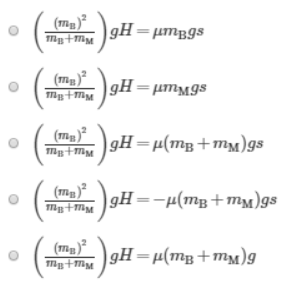# Problem: An iron block with mass mB slides down a frictionless hill of height H. At the base of the hill, it collides with and sticks to a magnet with mass mM.Now assume that the two masses continue to move at the speed v from Part A until they encounter a rough surface. The coefficient of friction is μ. If the blocks come to rest after a distance s, which of the following equations would you use to find s?

###### FREE Expert Solution

Take the speed of the block at the base of the hill to be v.

89% (76 ratings)###### Problem Details

An iron block with mass mB slides down a frictionless hill of height H. At the base of the hill, it collides with and sticks to a magnet with mass mM.

Now assume that the two masses continue to move at the speed v from Part A until they encounter a rough surface. The coefficient of friction is μ. If the blocks come to rest after a distance s, which of the following equations would you use to find s?